# Algebra 1 : How to find direct variation

## Example Questions

2 Next →

### Example Question #11 : How To Find Direct Variation

The amount of money Billy earns is directly proportional to his hours worked.  Suppose he earns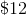every eight hours of work.  What is the minimum hours Billy must work in order to exceed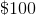?  Round to the nearest integer.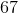Explanation:

Write the formula for direct proportionality.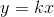Let: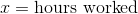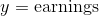Substitute twelve dollars and eight hours into this equation to solve for.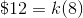Divide by eight on both sides.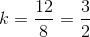Substituteback into the formula.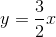To find out the minimum number of hours Billy must work to make, substituteintoand solve for.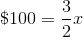Multiply by two thirds on both sides.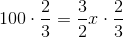Simplify both sides.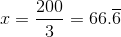Billy must work at leasthours to earn as much required.

2 Next →

### All Algebra 1 Resources